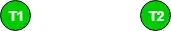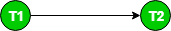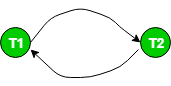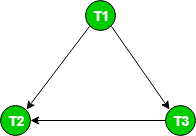# Precedence Graph for Testing Conflict Serializability in DBMS

A Precedence Graph or Serialization Graph is used commonly to test the Conflict Serializability of a schedule. It is a directed Graph (V, E) consisting of a set of nodes V = {T1, T2, T3……….Tn} and a set of directed edges E = {e1, e2, e3………………em}. The graph contains one node for each Transaction Ti. An edge ei is of the form Tj –> Tk where Tj is the starting node of ei and Tk is the ending node of ei. An edge ei is constructed between nodes Tj to Tk if one of the operations in Tj appears in the schedule before some conflicting operation in Tk. The Algorithm can be written as:

• Create a node T in the graph for each participating transaction in the schedule.
• For the conflicting operation read_item(X) and write_item(X) – If a Transaction Tj executes a read_item (X) after Ti executes a write_item (X), draw an edge from Ti to Tj in the graph.
• For the conflicting operation write_item(X) and read_item(X) – If a Transaction Tj executes a write_item (X) after Ti executes a read_item (X), draw an edge from Ti to Tj in the graph.
• For the conflicting operation write_item(X) and write_item(X) – If a Transaction Tj executes a write_item (X) after Ti executes a write_item (X), draw an edge from Ti to Tj in the graph.
• Schedule S is serializable if there is no cycle in the precedence graph.

If there is no cycle in the precedence graph, it means we can construct a serial schedule S’ which is conflict equivalent to schedule S. The serial schedule S’ can be found by Topological Sorting of the acyclic precedence graph. Such schedules can be more than 1. For example, Consider the schedule S:

` S: r1(x) r1(y) w2(x) w1(x) r2(y)`

## Creating Precedence Graph

Step 1: Make two nodes corresponding to Transaction T1 and T2.Step 1

Step 2: For the conflicting pair r1(x) w2(x), where r1(x) happens before w2(x), draw an edge from T1 to T2.Step 2

Step 3: For the conflicting pair w2(x) w1(x), where w2(x) happens before w1(x), draw an edge from T2 to T1.Step 3

Since the graph is cyclic, we can conclude that it is not conflict serializable to any schedule serial schedule. Let us try to infer a serial schedule from this graph using topological ordering. The edge T1–>T2 tells that T1 should come before T2 in the linear ordering. The edge T2 –> T1 tells that T2 should come before T1 in the linear ordering. So, we can not predict any particular order (when the graph is cyclic). Therefore, no serial schedule can be obtained from this graph.
Consider another schedule S1:

`S1: r1(x) r3(y) w1(x) w2(y) r3(x) w2(x)`Precedence Graph

The graph for this schedule is: Since the graph is acyclic, the schedule is conflict serializable. Performing Topological Sort on this graph would give us a possible serial schedule that is conflict equivalent to schedule S1. In Topological Sort, we first select the node with in-degree 0, which is T1. This would be followed by T3 and T2. So, S1 is conflict serializable since it is conflict equivalent to the serial schedule T1 T3 T2. Source: Operating Systems book, Silberschatz, Galvin and Gagne.

In DBMS, a precedence graph is used to test for conflict serializability, which is a property of a schedule that ensures that the transactions in the schedule can be executed in serial order without any conflicts. The precedence graph is a directed graph that represents the transaction dependencies in the schedule.

## What are the Steps to Construct a Precedence Graph?

Step 1: Draw a node for each transaction in the schedule.

Step 2: For each pair of conflicting operations (i.e., operations on the same data item by different transactions), draw an edge from the transaction that performed the first operation to the transaction that performed the second operation. The edge represents a dependency between the two transactions.

Step 3: If there are multiple conflicting operations between two transactions, draw multiple edges between the corresponding nodes.

Step 4: If there are no conflicting operations between two transactions, do not draw an edge between them.

Step 5: Once all the edges have been added to the graph, check if the graph contains any cycles. If the graph contains cycles, then the schedule is not conflict serializable. Otherwise, the schedule is conflict serializable.

The precedence graph provides a visual representation of the dependencies between transactions in a schedule and allows us to determine whether the schedule is a conflict serializable or not. By constructing the precedence graph, we can identify the transactions that have conflicts and reorder them to produce a conflict serializable schedule, which is a schedule that can be transformed into a serial schedule by swapping non-conflicting operations.

## Advantages of Precedence Graphs for Testing Conflict Serializability

• Simple to comprehend: Because precedence graphs show the connections between transactions visually, they are simple to comprehend.
• Quick analysis: You can rapidly ascertain whether or not a series of transactions can be conflict serialized by using precedence graphs.
• Finding anomalies: Anomalies like cycles or deadlocks that might not be seen right away might be found using precedence graphs.
• Assists with optimization: By identifying transactions that can be carried out in parallel, precedence graphs can be utilized to enhance a database system’s performance.

## Disadvantages of Precedence Graphs for Testing Conflict Serializability

• Complex for large systems: It can be challenging to discern dependencies between transactions in large database systems due to the complexity of precedence graphs.
• Potential for inaccurate results: It is possible that some conflicts between transactions will be unnoticed by precedence graphs.
• Require Manual efforts: Building precedence graphs by hand can be labor-intensive and time-consuming, particularly in the case of big systems.
• Limited applicability: Data races and deadlocks cannot be detected with precedence graphs; they are only useful for assessing conflict serializability.

## Conclusion

The precedence graph is one of the most important tools in database management. It is used to test the conflict serialization of transaction schedules. The graph identifies cycles in the execution sequence of transactions to determine if the schedule is conflict serializable.

### Q.1: What is the Precedence Graph method in DBMS?

The Precedence Graph Method in DBMS is generally used for testing the Conflict Serializability of the Schedule that results in setting the Concurrency Control in DBMS.